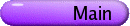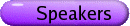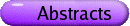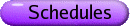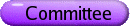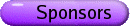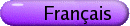Doctoral Prize Lecture VASILISA SHRAMCHENKO, Concordia University, Montreal, Canada Frobenius structures on Hurwitz spaces [PDF] Frobenius manifolds were introduced by Dubrovin to give a geometric reformulation of the WDVV (Witten-Dijkgraaf-Verlinde-Verlinde) system of differential equations, which describes deformations of topological field theories. The Frobenius manifold structures appear in various actively studied branches of mathematics revealing remarkable relationships between different theories such as the theory of Gromov-Witten invariants, singularity theory, differential geometry of the orbit spaces of reflection groups, and the Hamiltonian theory of integrable hierarchies. Frobenius structures on Hurwitz spaces (moduli spaces of functions over Riemann surfaces) constitute an important class of Frobenius manifolds. These structures admit an explicit description in terms of meromorphic objects defined on a Riemann surface and therefore are useful in understanding properties of a generic Frobenius manifold. The first examples of Hurwitz Frobenius structures were discovered by Dubrovin. In this talk I will discuss some examples of Frobenius manifolds. Then I will describe new Frobenius structures on Hurwitz spaces, namely the "real doubles" and "deformations" of Dubrovin's construction. The "real doubles" are Frobenius structures on a Hurwitz space considered as a real manifold. There are two "real doubles" for each Hurwitz Frobenius manifold. The "deformations" are obtained by introducing parameters into Dubrovin's construction. In a certain limit, the deformations reduce to the original structures. Reproducing kernels on Riemann surfaces (the Schiffer and Bergman kernels) are the main tools of our construction.